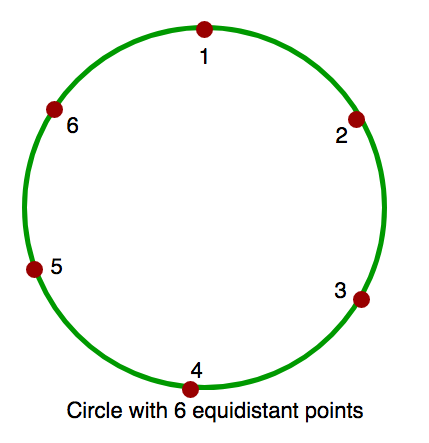Related Articles

# Count of obtuse angles in a circle with ‘k’ equidistant points between 2 given points

• Difficulty Level : Medium
• Last Updated : 16 Apr, 2021

A circle is given with k equidistant points on its circumference. 2 points A and B are given in the circle. Find the count of all obtuse angles (angles larger than 90 degree) formed from /_ACB, where C can be any point in circle other than A or B.
Note :
A and B are not equal.
A < B.
Points are between 1 and K(both inclusive).Examples :

```Input : K = 6, A = 1, B = 3.
Output : 1
Explanation : In the circle with 6
equidistant points, when C = 2 i.e.
/_123, we get obtuse angle.

Input : K = 6, A = 1, B = 4.
Output : 0
Explanation : In this circle, there
is no such C that form an obtuse angle.```

It can be observed that if A and B have equal elements in between them, there can’t be any C such that ACB is obtuse. Also, the number of possible obtuse angles are the smaller arc between A and B.
Below is the implementation :

## C++

 `// C++ program to count number of obtuse``// angles for given two points.``#include ``using` `namespace` `std;` `int` `countObtuseAngles(``int` `a, ``int` `b, ``int` `k)``{``    ``// There are two arcs connecting a``    ``// and b. Let us count points on``    ``// both arcs.``    ``int` `c1 = (b - a) - 1;``    ``int` `c2 = (k - b) + (a - 1);` `    ``// Both arcs have same number of``    ``// points``    ``if` `(c1 == c2)``        ``return` `0;` `    ``// Points on smaller arc is answer``    ``return` `min(c1, c2);``}` `// Driver code``int` `main()``{``    ``int` `k = 6, a = 1, b = 3;``    ``cout << countObtuseAngles(a, b, k);``    ``return` `0;``}`

## Java

 `// Java program to count number of obtuse``// angles for given two points``class` `GFG {` `    ``static` `int` `countObtuseAngles(``int` `a,``                                 ``int` `b, ``int` `k)``    ``{` `        ``// There are two arcs connecting a``        ``// and b. Let us count points on``        ``// both arcs.``        ``int` `c1 = (b - a) - ``1``;``        ``int` `c2 = (k - b) + (a - ``1``);` `        ``// Both arcs have same number of``        ``// points``        ``if` `(c1 == c2)``            ``return` `0``;` `        ``// Points on smaller arc is answer``        ``return` `min(c1, c2);``    ``}` `    ``// Driver Program to test above function``    ``public` `static` `void` `main(String arg[])``    ``{` `        ``int` `k = ``6``, a = ``1``, b = ``3``;``        ``System.out.print(countObtuseAngles(a, b, k));``    ``}``}` `// This code is contributed by Anant Agarwal.`

## Python

 `# C++ program to count number of obtuse``# angles for given two points.` `def` `countObtuseAngles( a, b, k):``    ``# There are two arcs connecting a``    ``# and b. Let us count points on``    ``# both arcs.``    ``c1 ``=` `(b ``-` `a) ``-` `1``    ``c2 ``=` `(k ``-` `b) ``+` `(a ``-` `1``)`` ` `    ``# Both arcs have same number of``    ``# points``    ``if` `(c1 ``=``=` `c2):``       ``return` `0``     ` `    ``# Points on smaller arc is answer``    ``return` `min``(c1, c2)`` ` `# Driver code``k, a, b ``=` `6``, ``1``, ``3``print` `countObtuseAngles(a, b, k)` `# This code is contributed by Sachin Bisht`

## C#

 `// C# program to count number of obtuse``// angles for given two points``using` `System;` `class` `GFG {` `    ``static` `int` `countObtuseAngles(``int` `a,``                           ``int` `b, ``int` `k)``    ``{` `        ``// There are two arcs connecting``        ``// a and b. Let us count points``        ``// on both arcs.``        ``int` `c1 = (b - a) - 1;``        ``int` `c2 = (k - b) + (a - 1);` `        ``// Both arcs have same number``        ``// of points``        ``if` `(c1 == c2)``            ``return` `0;` `        ``// Points on smaller arc is``        ``// answer``        ``return` `Math.Min(c1, c2);``    ``}` `    ``// Driver Program to test above``    ``// function``    ``public` `static` `void` `Main()``    ``{` `        ``int` `k = 6, a = 1, b = 3;``        ` `        ``Console.WriteLine(``           ``countObtuseAngles(a, b, k));``    ``}``}` `// This code is contributed by vt_m.`

## PHP

 ``

## Javascript

 ``

Output :

`1`

This article is contributed by Rohit Thapliyal. If you like GeeksforGeeks and would like to contribute, you can also write an article using contribute.geeksforgeeks.org or mail your article to contribute@geeksforgeeks.org. See your article appearing on the GeeksforGeeks main page and help other Geeks.